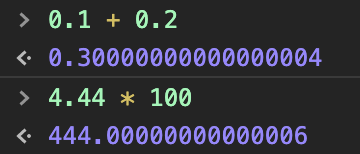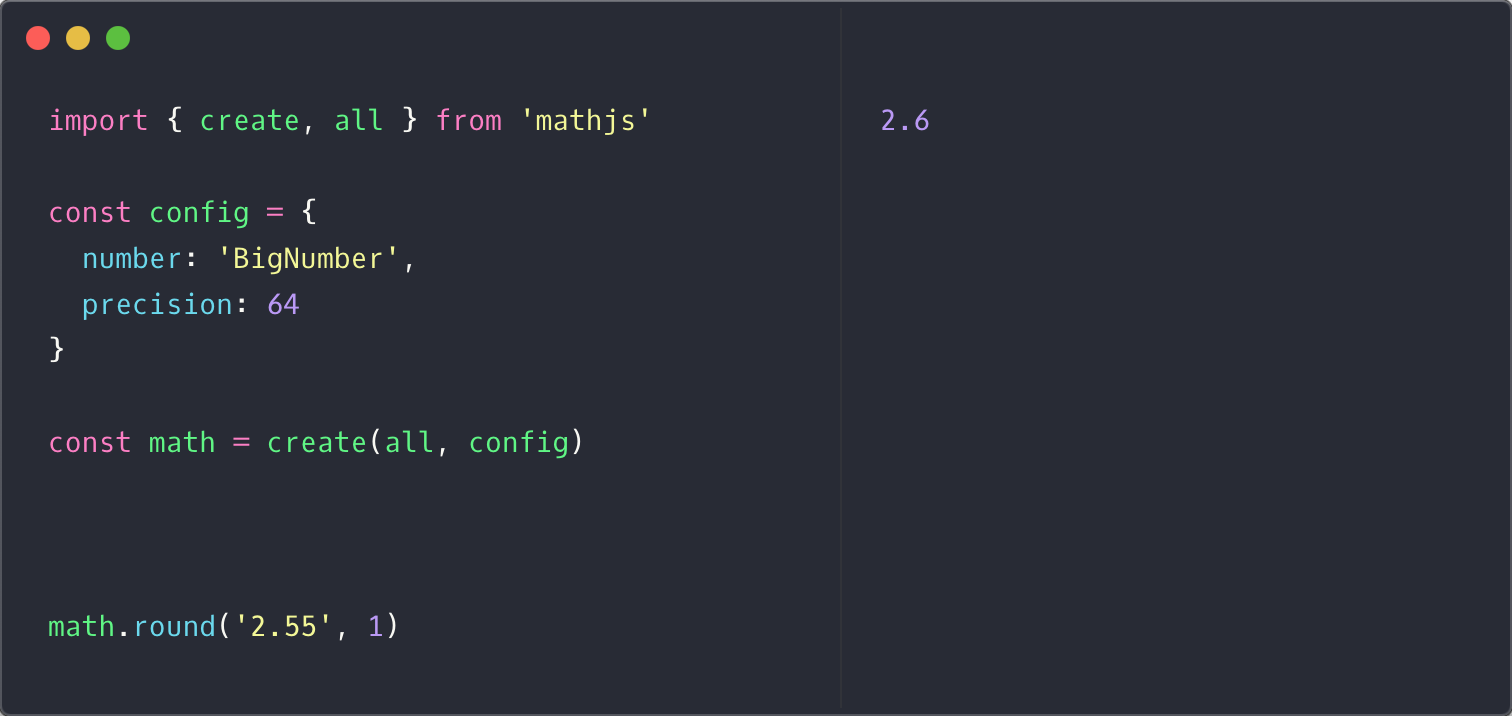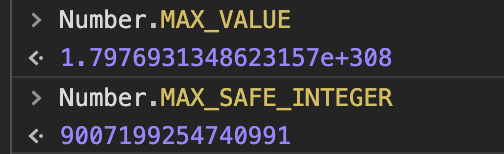JavaScript 安全计算
•   更新：2020-09-17 18:02:17
•   首发：2020-08-07 19:26:16
•   教程
•   3017“为什么JS中计算0.1加0.2的时候不等于0.3”，“为什么JS中计算4.44乘以100不等于444”，这类问题在诸多程序员岗位的面试中很常见。各个语言也提供了相应的数学库解决计算精度的问题。

Math.js是一个用于JavaScript和Node.js的高级数学计算函数库。它内置大量的函数和常量，具有支持符号计算的表达式解析器，并提供了一个集成的解决方案用于处理不同的数据类型，例如numbers、 big numbers、 complex numbers、 fractions、 units和matrices。强大且易于使用。

# 特征

• 支持数字，大数，复数，分数，单位，字符串，数组和矩阵。
• 与JavaScript的内置Math库兼容。
• 包含一个灵活的表达式解析器。
• 进行符号计算。
• 带有大量内置函数和常量。
• 也可以用作命令行应用程序。
• 在任何JavaScript引擎上运行。
• 很容易扩展。
• 开源。

# 安装

``````
npm install mathjs
``````

# 示例

## 常规计算（加减乘除）

``````
import { create, all } from 'mathjs'

const config = {

number: 'BigNumber',

precision: 64

}

const math = create(all, config)

math.evaluate('4.44 * 100')

math.evaluate('0.1 + 0.2')
``````## 四舍五入

``````NumberObject.toFixed(num)
``````### Round

#### 句法

``````math.round(x)
math.round(x, n)
``````

#### 参数

`x` number| BigNumber | Fraction | Complex | Array | Matrix 四舍五入
`n` number| BigNumber | Array 小数位数默认值：0。

#### 返回值

number| BigNumber | Fraction | Complex | Array | Matrix 取整值

#### 例子

``````math.round(3.2)              // returns number 3
math.round(3.8)              // returns number 4
math.round(-4.2)             // returns number -4
math.round(-4.7)             // returns number -5
math.round(math.pi, 3)       // returns number 3.142
math.round(123.45678, 2)     // returns number 123.46

const c = math.complex(3.2, -2.7)
math.round(c)                // returns Complex 3 - 3i

math.round([3.2, 3.8, -4.7]) // returns Array [3, 4, -5]
```````math.js`更多方法：https://mathjs.org/docs/reference/functions.html

# 取整

``````parseInt(string, radix)
``````

`parseInt(0.0000001)`的结果是`1`• 如果 string 以 "0x" 开头，parseInt() 会把 string 的其余部分解析为十六进制的整数。
• 如果 string 以 0 开头，那么 ECMAScript v3 允许 parseInt() 的一个实现把其后的字符解析为八进制或十六进制的数字。
• 如果 string 以 1 ~ 9 的数字开头，parseInt() 将把它解析为十进制的整数。

`>>>`是无符号位移。所以`>>> 0` 在JS里面是确保数字有意义的判断方法。

# 安全值与最大数

`1.7976931348623157e+308`，这个数字究竟意味着什么？`Number.MAX_SAFE_INTEGER` 是js里`整数`的安全的最大值，由于 js 用的是` IEEE 754 双精度浮点`，可以安全地表示 `[ -2^53+1 , 2^53-1 ] `这个范围。

`Number.MAX_VALUE` 属性是 JavaScript 中可表示的最大的数。

上一篇 (PHP 获取客户端真实IP地址)